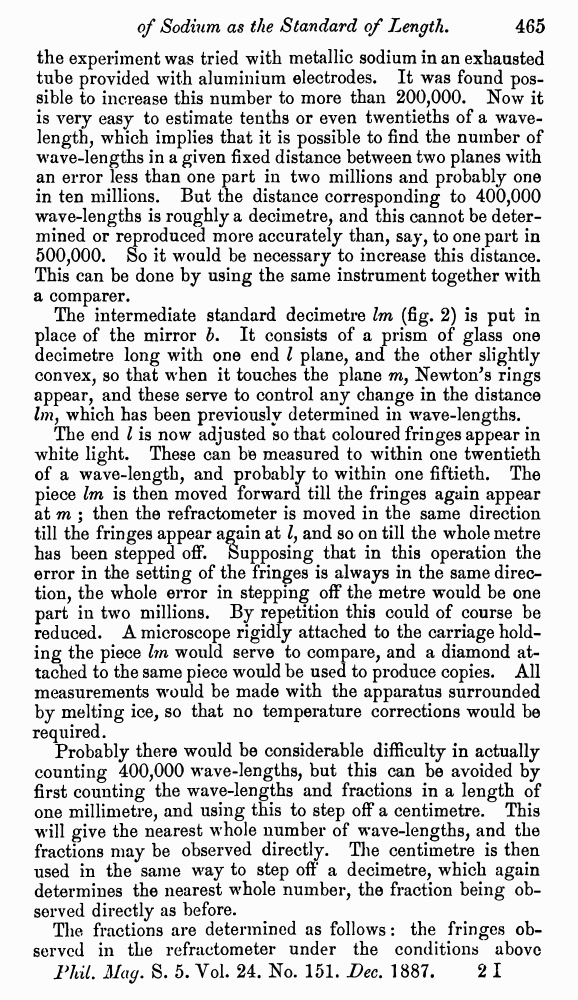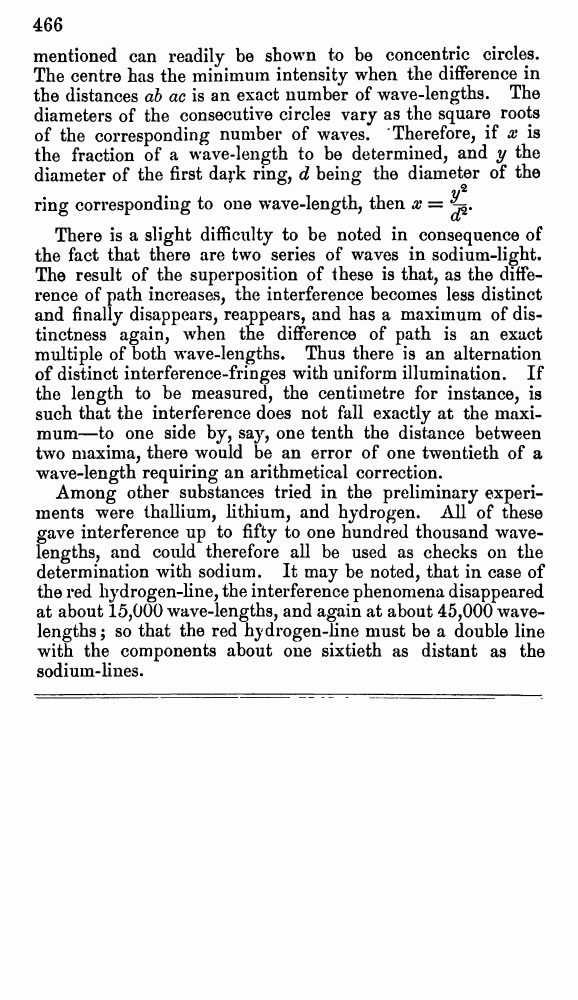# A. Michelson and E. Morley. On the Relative Motion of the Earth and the Luminiferous Æther. // Phil. Mag. S. 5. Vol. 24. No. 151. Dec. 1887.

В начало   Другие форматы   <<<     Страница 465   >>>449  450  451  452  453  454  455  456  457  458  459  460  461  462  463  464  465 466 the experiment was tried with metallic sodium in an exhausted tube provided with aluminium electrodes. It was found possible to increase this number to more than 200,000. Now it is very easy to estimate tenths or even twentieths of a wavelength, which implies that it is possible to find the number of wave-lengths in a given fixed distance between two planes with an error less than one part in two millions and probably one in ten millions. But the distance corresponding to 400,000 wave-lengths is roughly a decimetre, and this cannot be determined or reproduced more accurately than, say, to one part in 500,000. So it would be necessary to increase this distance. This can be done by using the same instrument together with a comparer. The intermediate standard decimetre lm (fig. 2) is put in place of the mirror b. It consists of a prism of glass one decimetre long with one end l plane, and the other slightly convex, so that when it touches the plane m, Newton's rings appear, and these serve to control any change in the distance which has been previously determined in wave-lengths. The end l is now adjusted so that coloured fringes appear in white light. These can be measured to within one twentieth of a wave-length, and probably to within one fiftieth. The piece lm is then moved forward till the fringes again appear at m ; then the refractometer is moved in the same direction till the fringes appear again at l, and so on till the whole metre has been stepped off. Supposing that in this operation the error in the setting of the fringes is always in the same direction, the whole error in stepping off the metre would be one part in two millions. By repetition this could of course be reduced. A microscope rigidly attached to the carriage holding the piece lm would serve to compare, and a diamond attached to the same piece would be used to produce copies. All measurements would be made with the apparatus surrounded by melting ice, so that no temperature corrections would be required. Probably there would be considerable difficulty in actually counting 400,000 wave-lengths, but this can be avoided by first counting the wave-lengths and fractions in a length of one millimetre, and using this to step off a centimetre. This will give the nearest whole number of wave-lengths, and the fractions may be observed directly. The centimetre is then used in the same way to step off a decimetre, which again determines the nearest whole number, the fraction being observed directly as before. The fractions are determined as follows : the fringes observed in the refractometer under the conditions above mentioned can readily be shown to be concentric circles. The centre has the minimum intensity when the difference in the distances ab ac is an exact number of wave-lengths. The diameters of the consecutive circles vary as the square roots of the corresponding number of waves. Therefore, if x is the fraction of a wave-length to be determined, and y the diameter of the first dark ring, d being the diameter of the ring corresponding to one wave-length, then There is a slight difficulty to be noted in consequence of the fact that there are two series of waves in sodium-light. The result of the superposition of these is that, as the difference of path increases, the interference becomes less distinct and finally disappears, reappears, and has a maximum of distinctness again, when the difference of path is an exact multiple of both wave-lengths. Thus there is an alternation of distinct interference-fringes with uniform illumination. If the length to be measured, the centimetre for instance, is such that the interference does not fall exactly at the maximum—to one side by, say, one tenth the distance between two maxima, there would be an error of one twentieth of a wave-length requiring an arithmetical correction. Among other substances tried in the preliminary experiments were thallium, lithium, and hydrogen. All of these gave interference up to fifty to one hundred thousand wavelengths, and could therefore all be used as checks on the determination with sodium. It may be noted, that in case of the red hydrogen-line, the interference phenomena disappeared at about 15,000 wave-lengths, and again at about 45,000 wavelengths; so that the red hydrogen-line must be a double line with the components about one sixtieth as distant as the sodium-lines.